# Texas Go Math Kindergarten Lesson 9.4 Answer Key Compose and Decompose Numbers Up to 5

Refer to our Texas Go Math Kindergarten Answer Key Pdf to score good marks in the exams. Test yourself by practicing the problems from Texas Go Math Kindergarten Lesson 9.4 Answer Key Compose and Decompose Numbers Up to 5.

## Texas Go Math Kindergarten Lesson 9.4 Answer Key Compose and Decompose Numbers Up to 5

Unlock the Problem

DIRECTIONS: Hannah has 4 counters. How many red and yellow counters could she have? Place the red counters in the five frames. Trace and color them. Write the number. Trace the plus symbol. Place the yellow counters in the five frames. Trace and color them. Write the number. Write the number to show how many counters in all.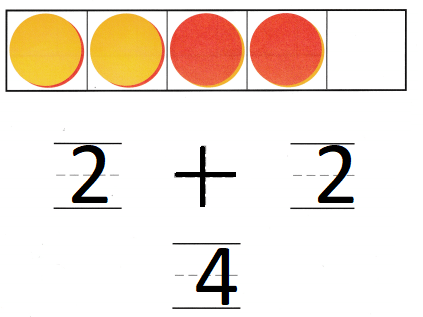Explanation:
The number of red counters are 2
the number of yellow counters are 2
Total number of counters 4
4 = 2 + 2

Try Another Problem

DIRECTIONS: 1-2. Listen to the problem. Draw and color counters to solve the problem. Write the numbers and trace the symbol.

Question 1.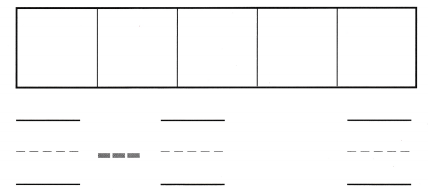Explanation:
The number of red counters are 2
the number of yellow counters are 3
Total number of counters 5
5 – 3 = 2

Question 2.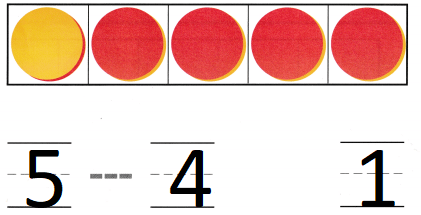Explanation:
The number of red counters are 4
the number of yellow counters are 1
Total number of counters 5
5 – 4 = 1

Share and Show

DIRECTIONS: 3-4. Listen to the problem. Draw and color counters to solve the problem. Write the numbers and trace the symbol.

Question 3.Explanation:
The number of red counters are 1
the number of yellow counters are 4
Total number of counters 5
4 + 1 = 5

Question 4.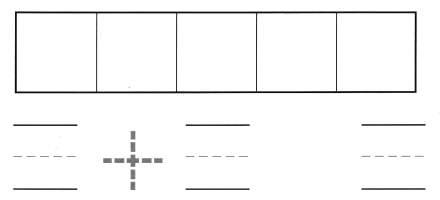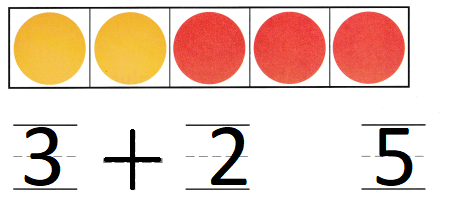Explanation:
The number of red counters are 3
the number of yellow counters are 2
Total number of counters 5
3 + 2 = 5Explanation:
Neeta has  three red and 2 yellow counters
so, Neeta has 5 counters in all.
The number of red counters are 3
the number of yellow counters are 2
Total number of counters 5
3 + 2 = 5

DIRECTIONS: Listen to the problem. Choose the correct answer. 5. The soccer team has 3 blue soccer balls. It also has 2 yellow soccer balls. How many soccer balls are there in all? 6. There are 3 counters. 2 are yellow. The rest are red. How many counters are red? 7. Which math statement describes the cubes?

Question 5.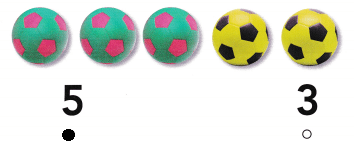Explanation:
The soccer team has 3 blue soccer balls. It also has 2 yellow soccer balls.
3 + 2 = 5
5  soccer balls are there in all

Question 6.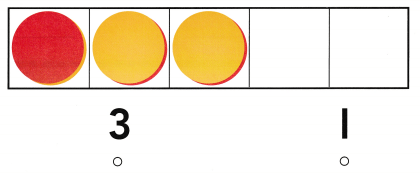Explanation:
There are 3 counters. 2 are yellow.
The rest are red.
3 – 2 = 1
so,1 counter is red

Question 7.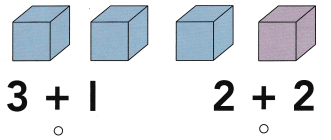Explanation:
There are 3 blue cubes and 1 purple cube
3 + 1 = 4
is the correct statement that matches the cube.

### Texas Go Math Kindergarten Lesson 9.4 Homework and Practice Answer Key

DIRECTIONS: 1-2. Draw 5 counters. Color some of the yellow. Write the number. Trace the symbol. Color the rest of the counters red. Write the number. Write the number to show how many counters in all.

Question 1.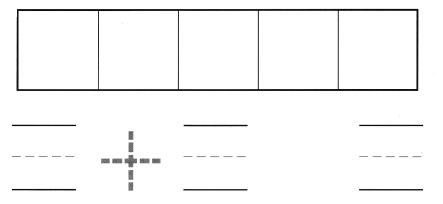Explanation:
The number of red counters are 3
the number of yellow counters are 2
Total number of counters 5
3 + 2 = 5

Question 2.Explanation:
The number of red counters are 1
the number of yellow counters are 4
Total number of counters 5
4 + 1 = 5

DIRECTIONS: Choose the correct answer. 3. Dean has 2 yellow hats. He also has two purple hats. How many hats does he have in all? 4. There are 4 counters. 1 is red. The rest are yellow. How many counters are yellow? 5. Which math statement describes the cubes?

Question 3.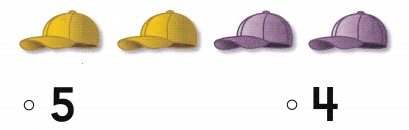Explanation:
Dean has 2 yellow hats. He also has two purple hats.
2 + 2 = 4
4 hats that he have in all

Question 4.Explanation:
There are 4 counters. 1 is red.
The rest are yellow.
4 – 1 = 3
so, three counters are yellow

Question 5.